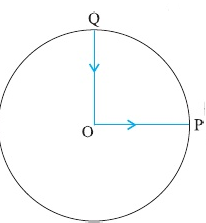## Question

### Solution

Correct option is

None fo these

Distance travelled in the last two seconds = area of triangle BDCThe distance travelled in seven seconds = area of triangle OAE + area of rectangle ABDE + area of triangle BDC= 90 m.

#### SIMILAR QUESTIONS

Q1

A freely falling body, falling from a tower of height h covers a distanceh/2 in the last second of its motion. The height of the tower is (take g = 10 ms–2) nearly

Q2A cyclist starts from the centre O of a circular track of radius r = 1 km, reaches the edge P of the track and then cycles along circumference and stops at point Q. if the total time take is 10 min, what is the average velocity of the cyclist?

Q3

A stone falls from rest. The distance covered by the stone in the last second of its motion equals the distance covered by it during the first three seconds of its motion. How long does the stone take to reach the ground?

Q4

Two balls of masses m1 and m2 are thrown vertically upward with the speed u. If air resistance is neglected, they will pass through their point of projection in the downward direction with a speed

Q5

A train covers half of its journey with a speed of 20 ms–1 and the other half with a speed of 30 ms–1. The average speed of the train during the whole journey is

Q6

The displacement y (in metres) of a body varies with time t (in seconds) asHow long does the body take to come to rest?

Q7

A body goes from A to B with a velocity of 40 kmh–1 and returns from Bto A with a velocity of 60 kmh–1. What is the average velocity of the body during the whole journey?

Q8

The area under the velocity-time graph between any two instants t = t1and t = t2 gives the distance covered in timeQ9

A particle is given a displacement of 4 m in the x-y plane. If the x-component of the displacement vector is 2 m, the y-component will be